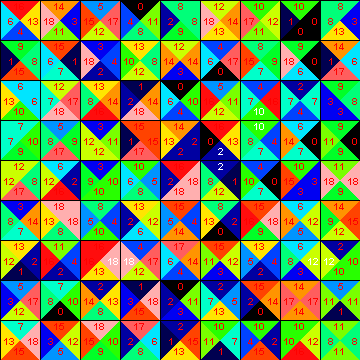### Problem Statement

In this problem you will solve a puzzle by placing tiles on a square board. You need to place all the tiles on the board, and can place each tile only once on the board. Each tile is square and contains four edges. Tiles can be placed rotated on the board. Your goal is to place all the tiles on the board such that the number of matching tile edges is maximized.

### Implementation Details

The board contains N rows and N columns. You are given a list of N*N tiles. You need to place all of these tiles on the board. The color of each edge of a tile will be selected from C distinct colors.

Your code should implement one method solvePuzzle. The size of the board are given to you in N. String[] tiles contains the description of the tiles that need to be placed on the board; each String contains the definition of one tile. Each element of tiles is formatted as "N E S W". Where N corresponds to the color of the edge that face north, E corresponds to the color of the edge that faces east, S? South and W- West. Colors are described by an integral number.

You must return a list of size N*N that specifies the placement of the tiles. Each element of your return should be formatted as "TILE ROTATION". The ith element in your return should correspond to the tile placed at column (i mod N) and row (i div N). "TILE" should correspond to the index of the tile as received in String[] tiles. "ROTATION" gives the rotation of the tile which can be 0 = No rotation, 1 = 90 degrees clockwise rotation, 2 = 180 degrees rotation, 3 = 270 degrees clockwise rotation.

### Scoring

Your score for a test case will be the number of matching edges divided by (N*(N-1)*2). The maximum score for a single test case is 1. An invalid return of any kind will result in zero absolute score for that test case. If you place the same tile more than once on the board, you will get a zero score. If you don?t place all the tiles on the board, you will get a zero score. Your overall score will be the sum of your scores over all test cases.

### Test Case Generation

The size of the board is chosen between 8 and 20, and the number of colors to use is chosen between 10 and 30. The board is randomly filled with tiles where every neighboring edge will match colors. See visualizer source code for more detail. The tiles are then extracted from the board and given to you in a random order and each tile adjusted with a random rotation.

### Visualizer

A visualizer is available for offline testing.

### Definition

 Class: TilesPuzzle Method: solvePuzzle Parameters: int, String[] Returns: String[] Method signature: String[] solvePuzzle(int N, String[] tiles) (be sure your method is public)

### Notes

-N will be chosen uniformly between 8 and 20 inclusively.
-C will be chosen uniformly between 10 and 30 inclusively.
-A perfect solution for each test case does exist.
-For more implementation details see the visualizer source.
-The memory limit is 1024 MB and the time limit is 20 seconds (which includes only time spent in your code).
-There are 10 example test cases and 100 full submission test cases.

### Examples

0)

 `"1"`
```Returns: "seed = 1
N = 9
C = 19"```1)

 `"2"`
```Returns: "seed = 2
N = 8
C = 22"```2)

 `"3"`
```Returns: "seed = 3
N = 19
C = 22"```3)

 `"4"`
```Returns: "seed = 4
N = 11
C = 13"```
4)

 `"5"`
```Returns: "seed = 5
N = 16
C = 10"```
5)

 `"6"`
```Returns: "seed = 6
N = 19
C = 22"```
6)

 `"7"`
```Returns: "seed = 7
N = 18
C = 12"```
7)

 `"8"`
```Returns: "seed = 8
N = 8
C = 16"```
8)

 `"9"`
```Returns: "seed = 9
N = 14
C = 25"```
9)

 `"10"`
```Returns: "seed = 10
N = 17
C = 20"```

#### Problem url:

http://www.topcoder.com/stat?c=problem_statement&pm=10474

#### Problem stats url:

http://www.topcoder.com/tc?module=ProblemDetail&rd=13897&pm=10474

Unknown

Search